# Brelot harmonic space

Roughly speaking, a Brelot harmonic space is a locally compact space endowed with an additional structure making it possible to study notions known from classical potential theory, such as harmonic and hyperharmonic functions, potentials, minimum principle, the Dirichlet problem, harmonic measure, balayage, fine topology, Martin compactification, etc. Standard examples are provided by elliptic partial differential equations in Euclidean spaces or on manifolds or by harmonic functions on a Riemann surface. (Cf. also Harmonic space; Potential theory, abstract.)

Letbe a locally compact, locally connected topological space and let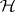be a sheaf of vector spaces of real-valued continuous functions. This means that to every non-empty open set, a vector spaceconsisting of continuous functions onis associated in such a way that: i) if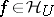,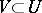is a non-empty open set, then the restriction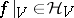; and ii) if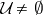is a family of non-empty open sets with unionandis a function onsuch that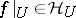for every, then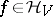. (The elements of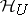are called harmonic functions on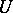with respect to; cf. also Harmonic function.)

The sheafis called a Brelot harmonic structure (the terminology from [a1]) if the following three axioms hold:

I)is not degenerate, i.e., for everythere exists an open neighbourhoodofand a strictly positive function;

II) (the base axiom) the topology ofhas a basis consisting of regular sets. (Here, a set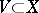is said to be regular (with respect to) if the Dirichlet problem onis solvable in the following sense: For every real-valued continuous functionon the boundary of, there exists a uniquely determined harmonic functionwhich extendscontinuously. Furthermore,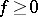implies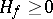.)

III) the Brelot convergence axiom: for every increasing sequence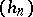of harmonic functions on a domain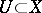one has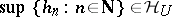, provided that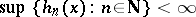for some.

The pair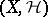is then called a Brelot harmonic space. (These spaces were introduced by M. Brelot in 1957; see [a3]. Later on, more general axiomatic settings for potential theory were developed, mainly by H. Bauer, C. Constantinescu and A. Cornea, J. Bliedtner and W. Hansen, N. Boboc, Gh. Bucur and Cornea; see [a1] and [a2].)

Results of J.M. Bony (see e.g. [a1]) show that, in a sense, the theory of Brelot harmonic spaces is close to potential theory for second-order partial differential equations of elliptic type (cf. also Potential theory, abstract). A great deal of results known from classical potential theory (which corresponds to the Laplace equation) can be obtained in the framework of Brelot harmonic spaces; sometimes additional hypotheses are imposed. Moreover, there is an important and deep connection between a class of Markov processes and Brelot harmonic spaces (see [a1] and [a2] and Markov process).